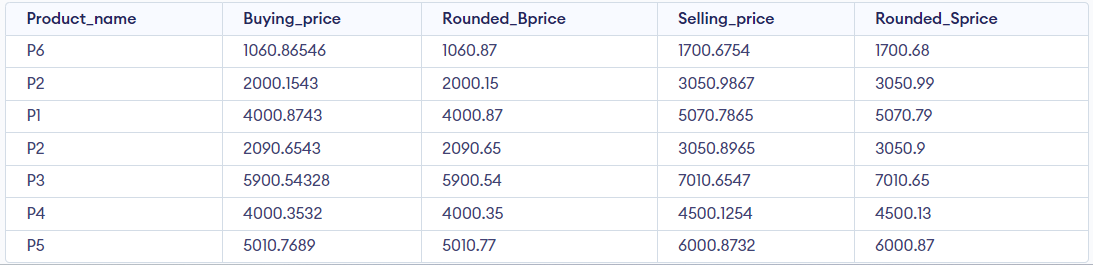GeeksforGeeks App
Open AppBrowser
Continue

The ROUND() function in MySQL is used to round a number to a specified number of decimal places. If no specified number of decimal places is provided for round-off, it rounds off the number to the nearest integer.

Syntax

ROUND(X, D)

Parameter Explanation

This method accepts two parameters in the syntax, as mentioned above and described below –

• X: The number which to is rounded.
• D: Number of decimal places up to which the given number is to be rounded. It is optional. If not given it round off the number to the closest integer. If it is negative, then the number is rounded to the left side of the decimal point.
• Returns: It returns the number after rounding to the specified places.

Example-1

Rounding off a number when D is not specified.

Rounding a Negative number.

Query:

`SELECT ROUND(-10.11) AS Rounded_Number;`

Output: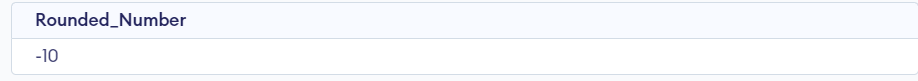Rounding a Positive number.

Query:

`SELECT ROUND(100.61) AS Rounded_Number;`

Output: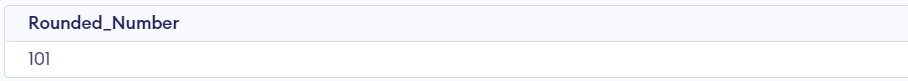Example-2

Rounding off a number when D is negative(-ve).

Rounding a Negative number.

Query:

`SELECT ROUND(-1567.1100, -3) AS Rounded_Number;`

Output: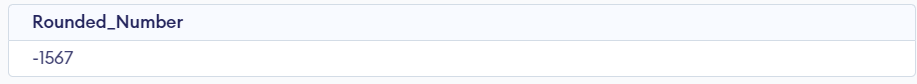Rounding a Positive number.

Query:

`SELECT ROUND(1016.6089, -1) AS Rounded_Number;`

Output: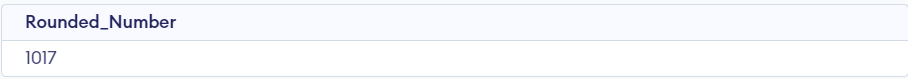Example-3

Rounding off a number when D is positive(+ve).

Rounding a Negative number up to 2 decimal places.

Query:

`SELECT ROUND(-1567.1160, 2) AS Rounded_Number;`

Output: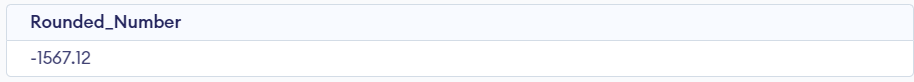Rounding a Positive number up to three decimal places.

Query:

`SELECT ROUND(1016.6019, 3) AS Rounded_Number;`

Output: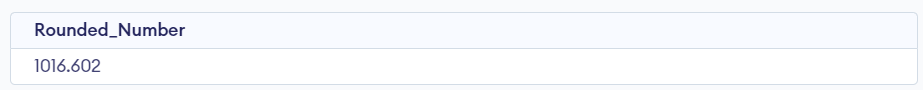Example-4

The ROUND Function can also be used to find the rounded values for the column data. In this example, we are going to find rounded values for the Price column. To demonstrate create a table named Product.

Query:

```CREATE TABLE Product(
Product_id INT AUTO_INCREMENT,
Product_name VARCHAR(100) NOT NULL,
Selling_price DECIMAL(13, 6) NOT NULL,
Selling_Date Date NOT NULL,
PRIMARY KEY(Product_id)
);```

Now insert some data into the Product table –

Query:

```INSERT INTO
VALUES
('P6', 1060.865460, 1700.675400, '2020-08-26'),
('P2', 2000.154300, 3050.986700, '2020-08-27'),
('P1', 4000.874300, 5070.786500, '2020-08-28'),
('P2', 2090.654300, 3050.896500, '2020-09-01'),
('P3', 5900.543280, 7010.654700, '2020-09-04'),
('P4', 4000.353200, 4500.125400, '2020-09-05'),
('P5', 5010.768900, 6000.873200, '2020-09-08');```

So, the Product Table is as –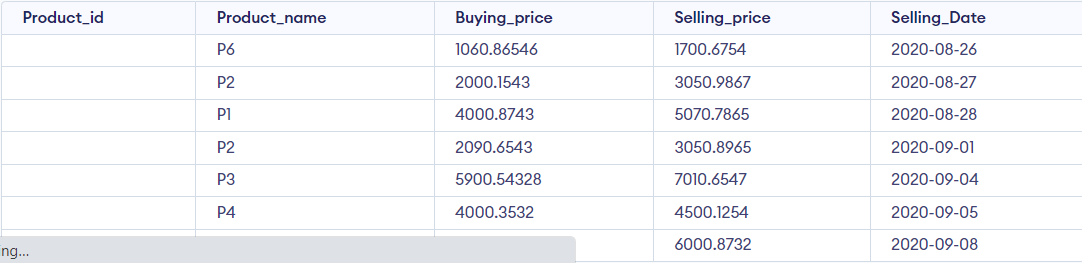Now, we are going to round off both Buying_price and Selling_price columns up to 2 decimal places.

Query:

```SELECT Product_name, Buying_price,